﻿ 新冠病毒DNA序列基于熵值的分布可视化 Novel Coronavirus DNA Sequence Visualization Based on Entropy Distribution

Hans Journal of Computational Biology
Vol. 11  No. 02 ( 2021 ), Article ID: 43003 , 9 pages
10.12677/HJCB.2021.112003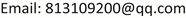Novel Coronavirus DNA Sequence Visualization Based on Entropy Distribution

Chen Yang, Jeffrey Zheng

School of Software, Yunnan University, Kunming YunnanReceived: May 1st, 2021; accepted: Jun. 2nd, 2021; published: Jun. 9th, 2021ABSTRACT

Novel coronavirus is officially named 2019-ncov in January 2020, and has not been well controlled until now. The DNA base sequence of avirus plays a decisive role in the character of avirus. This paper analyzes the differences between novel coronavirus DNA from four countries (China, America, Australia and Germany). The novel coronavirus DNA sequences from different countries were selected, and the visual analysis of the differences between four viruses was given in the form of information entropy, relative entropy and cross entropy.

Keywords:Novel Coronavirus, DNA Sequence, Entropy, Visualization Analysis1. 引言

2020年3月底，新冠病毒在我国得到了基本的控制，但全球的疫情远远没有结束，社会上也出现了各种关于病毒来自哪个国家的讨论，这时候利用科学的方法来辨识病毒在微观上的差异也显得尤为重要。

DNA的一级结构决定了基因的功能，欲想解释基因的生物学含义，首先必须知道其DNA顺序。

2. 研究结构

2.1. 信息熵

$H\left(U\right)=E\left[-\mathrm{log}{p}_{i}\right]=-{\sum }_{i=1}^{n}\mathrm{log}{p}_{i}$ (1)

2.2. 相对熵

$KL\left(P‖Q\right)=\underset{x\in X}{\sum }P\left(x\right)\mathrm{log}\frac{P\left(x\right)}{Q\left(x\right)}$ (2)

2.3. 交叉熵

$H\left(p,q\right)=\underset{x}{\sum }P\left(x\right)\mathrm{log}\left(\frac{1}{q\left(x\right)}\right)$ (3)

2.4. 新冠病毒DNA碱基序列

2.5. 研究方法与研究模块

2.5.1. 参数

2.5.2. 计量模块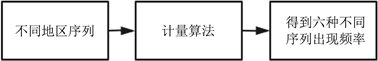Figure 1. Measuring module

2.5.3. 处理模块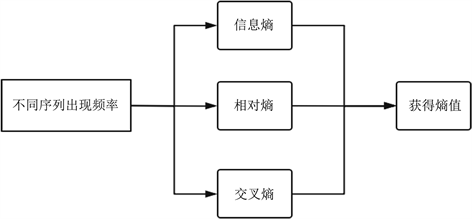Figure 2. Processing module

2.5.4. 可视化模块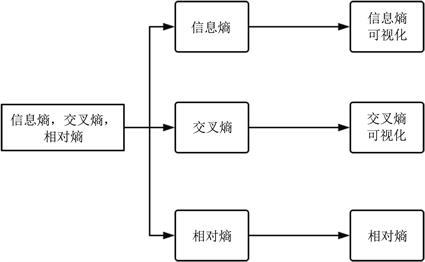Figure 3. Visualization module

3. 可视化结果分析

3.1. 四个地区，六种分布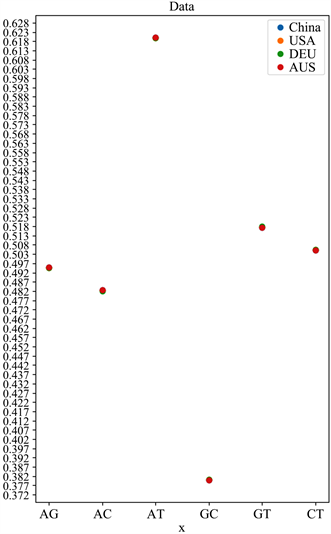Figure 4. Six kinds of sequence frequency distributionFigure 5. AG sequence frequency distributionFigure 6. AC sequence frequency distribution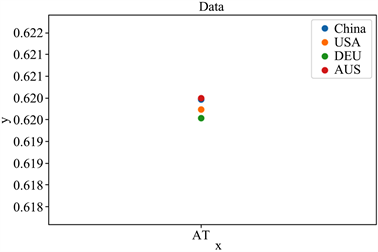Figure 7. AT sequence frequency distribution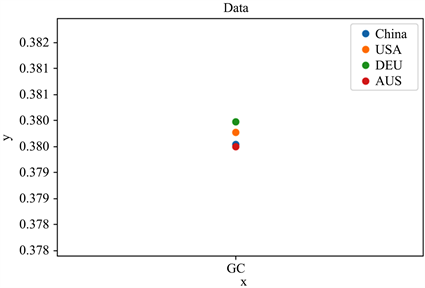Figure 8. GC sequence frequency distribution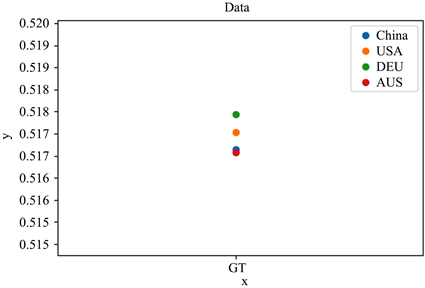Figure 9. GT sequence frequency distribution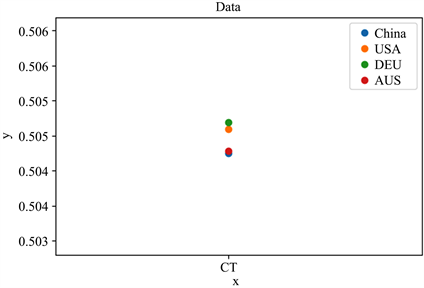Figure 10. CT sequence frequency distribution

3.2. 基因序列的信息熵

$H\left(x\right)=-\underset{i=1}{\overset{n}{\sum }}p\left({x}_{i}\right)\mathrm{log}p\left({x}_{i}\right)$ (4)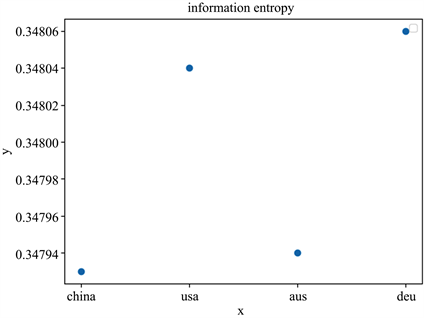Figure 11. Information entropy of four regions

3.3. 基因中AG分布的相对熵

$\begin{array}{c}DKL\left(p‖q\right)=-\int p\left(x\right)\mathrm{ln}q\left(x\right)-\left(-\int p\left(x\right)\mathrm{ln}p\left(x\right)\text{d}x\right)\\ =\underset{i=1}{\overset{n}{\sum }}p\left({x}_{i}\right)\mathrm{log}\left(\frac{p\left({x}_{i}\right)}{q\left({x}_{i}\right)}\right)\end{array}$ (5)

3.4. 基因中AG分布的交叉熵

$DKL_C=-\int p\left(x\right)\mathrm{ln}\left(q\left(x\right)\text{d}x\right)$ (6)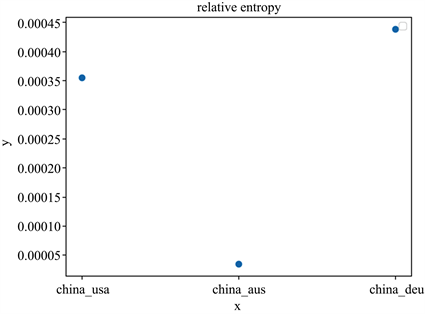Figure 12. The relative entropy of the AG distributionFigure 13. The cross entropy of the AG distribution

4. 总结

Novel Coronavirus DNA Sequence Visualization Based on Entropy Distribution[J]. 计算生物学, 2021, 11(02): 21-29. https://doi.org/10.12677/HJCB.2021.112003

1. 1. Kullback, S. and Leibler, R.A. (1951) On Information and Sufficiency. The Annals of Mathematical Statistics, 22, 79-86. https://doi.org/10.1214/aoms/1177729694

2. 2. Goodfellow, I., Bengio, Y. and Courville, A. (2016) Deep Learning (Vol. 1). MIT Press, Cambridge, 71-73.